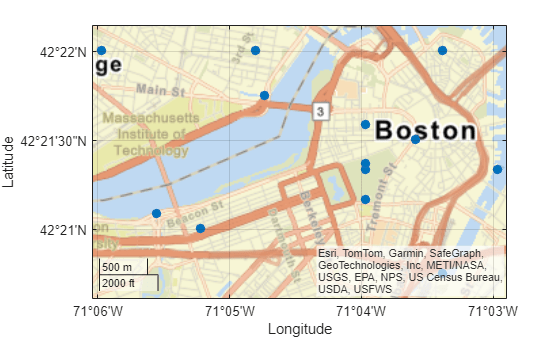geopointshape

Point shape in geographic coordinates

Description

A geopointshape object represents a point or multipoint in geographic coordinates. A multipoint is an individual point shape that contains a set of point locations.

To represent a point or multipoint in planar coordinates, use a mappointshape object instead.

Creation

To create geopointshape objects, either:

• Import point data in geographic coordinates as a geospatial table using the readgeotable function, and then query the Shape variable of the table.

• Use the geopointshape function (described here).

Description

example

shape = geopointshape(lat,lon) creates a geopointshape object or array of geopointshape objects with the specified latitude and longitude coordinates. The sizes of lat, lon, and the geopointshape object array shape match.

Input Arguments

expand all

Latitude coordinates, specified as a numeric array or a cell array of numeric arrays.

• Create a point by specifying a scalar, such as 39.

• Create a multipoint by specifying an array within a cell, such as {[38 -30 29]}.

• Create an array of points by specifying an array, such as [38 -30 29].

• Create an array of points and multipoints by specifying a cell array of numeric arrays, such as {39,[38 -30 29]}.

Create placeholders for points with missing data by including NaN values. The NaN values in lat must correspond to the NaN values in lon.

The size of lat must match the size of lon. For cell arrays, the size of the array in each cell of lat must match the size of the array in the corresponding cell of lon.

Data Types: double | cell

Longitude coordinates, specified as a numeric array or a cell array of numeric arrays.

• Create a point by specifying a scalar, such as -113.

• Create a multipoint by specifying an array within a cell, such as {[-66 -31 42]}.

• Create an array of points by specifying an array, such as [-66 -31 42].

• Create an array of points and multipoints by specifying a cell array of numeric arrays, such as {-113,[-66 -31 42]}.

Create placeholders for points with missing data by including NaN values. The NaN values in lat must correspond to the NaN values in lon.

The size of lat must match the size of lon. For cell arrays, the size of the array in each cell of lat must match the size of the array in the corresponding cell of lon.

Properties

expand all

Number of points, returned as an array of nonnegative integers.

For a geopointshape scalar, the value of NumPoints is 1 when the geopointshape object represents a single point and more than 1 when the object represents a multipoint.

For a geopointshape array, the size of NumPoints matches the size of the array.

Data Types: double

Latitude coordinates, specified as an array.

For a geopointshape scalar, the size of Latitude matches the value of NumPoints.

For a geopointshape array, the size of Latitude matches the size of NumPoints. If the array contains geopointshape objects with multipoints, then accessing the Latitude property of the array is not supported. Instead, access the Latitude property of individual objects within the array.

This property is read-only for arrays when any element of NumPoints is greater than 1.

Latitude and Longitude must be the same size.

Data Types: double

Longitude coordinates, specified as an array.

For a geopointshape scalar, the size of Longitude matches the value of NumPoints.

For a geopointshape array, the size of Longitude matches the size of NumPoints. If the array contains geopointshape objects with multipoints, then accessing the Longitude property of the array is not supported. Instead, access the Longitude property of individual elements within the array.

This property is read-only for arrays when any element of NumPoints is greater than 1.

Latitude and Longitude must be the same size.

Data Types: double

Geometric type, returned as "point".

Data Types: string

Coordinate system type, returned as "geographic".

Data Types: string

Geographic coordinate reference system (CRS), specified as a geocrs object. A geographic CRS consists of a datum (including its ellipsoid), prime meridian, and angular unit of measurement.

Examples

collapse all

Import a GPX file containing the coordinates of locations in Boston as a geospatial table. The GPX file represents the locations using points. Get information about the points by querying the Shape variable of the table.

GT.Shape
ans =
13×1 geopointshape array with properties:

NumPoints: [13×1 double]
Latitude: [13×1 double]
Longitude: [13×1 double]
Geometry: "point"
CoordinateSystemType: "geographic"
GeographicCRS: [1×1 geocrs]

Extract the latitude and longitude coordinates from the Shape variable. Display the locations on a road map.

lat = GT.Shape.Latitude;
lon = GT.Shape.Longitude;
geoscatter(lat,lon,"filled")
geobasemap("streets")Create an individual point as a geopointshape scalar. Specify the geographic CRS as the World Geodetic System of 1984, which has the EPSG code 4326.

point = geopointshape(39,-113);
g = geocrs(4326);
point.GeographicCRS = g
point =
geopointshape with properties:

NumPoints: 1
Latitude: 39
Longitude: -113
Geometry: "point"
CoordinateSystemType: "geographic"
GeographicCRS: [1x1 geocrs]

Create a multipoint as a geopointshape scalar.

multipoint = geopointshape({[38 -30 29]},{[-66 -31 42]});
multipoint.GeographicCRS = g
multipoint =
geopointshape with properties:

NumPoints: 3
Latitude: [38 -30 29]
Longitude: [-66 -31 42]
Geometry: "point"
CoordinateSystemType: "geographic"
GeographicCRS: [1x1 geocrs]

Create three individual points as a 1-by-3 geopointshape vector.

pointVector = geopointshape([38 -20 29],[-66 -31 42]);
pointVector.GeographicCRS = g
pointVector =
1x3 geopointshape array with properties:

NumPoints: [1 1 1]
Latitude: [38 -20 29]
Longitude: [-66 -31 42]
Geometry: "point"
CoordinateSystemType: "geographic"
GeographicCRS: [1x1 geocrs]

Create one individual point and one multipoint as a 1-by-2 geopointshape vector.

pointMultipoint = geopointshape({39,[38 -30 29]},{-113,[-66 -31 42]});
pointMultipoint.GeographicCRS = g
pointMultipoint =
1x2 geopointshape array with properties:

NumPoints: [1 3]
Geometry: "point"
CoordinateSystemType: "geographic"
GeographicCRS: [1x1 geocrs]

Topics

Introduced in R2021b

Mapping Toolbox Documentation